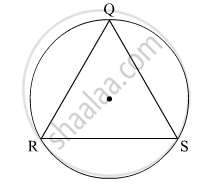Advertisement Remove all ads

# In the Given Figure, ∆Qrs is an Equilateral Triangle. Prove That, (1) Arc Rs ≅ Arc Qs ≅ Arc Qr (2) M(Arc Qrs) = 240°. - Geometry

Sum

In the given figure, ∆QRS is an equilateral triangle. Prove that,
(1) arc RS ≅ arc QS ≅ arc QR
(2) m(arc QRS) = 240°.Advertisement Remove all ads

#### Solution

(1) It is given that ∆QRS is an equilateral triangle.
∴ chord RS = chord QS = chord QR                                 (Sides of an equilateral triangle are equal)
⇒ m(arc RS) = m(arc QS) = m(arc QR)         .....(1)          (Corresponding arcs of congruent chords of a circle are congruent)
(2) m(arc RS) + m(arc QS) + m(arc QR) = 360º                      (Measure of a complete circle is 360º)
⇒ m(arc RS) + m(arc RS) + m(arc RS) = 360º                [Using (1)]
⇒ 3 × m(arc RS) = 360º
⇒ m(arc RS) = 120º
∴ m(arc RS) = m(arc QR) = 120º
Now,
m(arc QRS) = m(arc QR) + m(arc RS)
⇒ m(arc QRS) = 120º + 120º = 240º

Concept: Property of Sum of Measures of Arcs
Is there an error in this question or solution?
Advertisement Remove all ads

#### APPEARS IN

Advertisement Remove all ads
Advertisement Remove all ads
Share
Notifications

View all notifications

Forgot password?